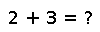# nthperm(p)

nthperm(p)

Return the `k` that generated permutation `p`. Note that `nthperm(nthperm([1:n], k)) == k` for `1 <= k <= factorial(n)`.

## Examples

Checking you are not a robot: